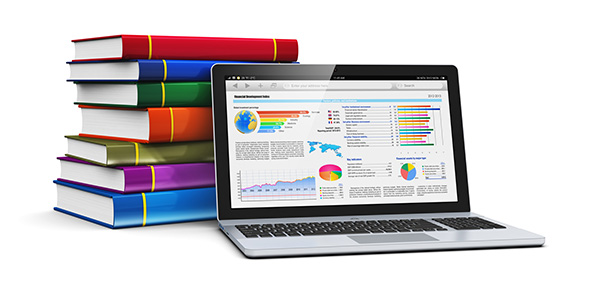# Metric Conversions

7 Questions | Total Attempts: 13SettingsNothing. . .

Related Topics
• 1.
How many gallons are in liter?
• 2.
How many pounds are in a kilogram?
• 3.
How many feet are in a meter?
• 4.
How many pounds are in a gram?
• 5.
How many kilometers are in a mile?
• 6.
If something is LARGER, and you're converting it to a SMALLER something, do you multiply or divide?
• 7.
If something is SMALLER and you're converting it to a LARGER something, do you multiply or divide? (Hint: This answer will be the opposite of you're answer to question 6)